# Topology

A valuation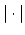on a field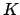induces a topology in which a basis for the neighborhoods of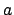are the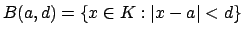for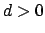.

Lemma 16.1.1   Equivalent valuations induce the same topology.

Proof. If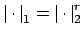, then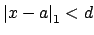if and only if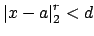if and only if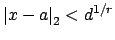so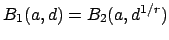. Thus the basis of open neighborhoods offor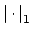and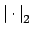are identical.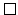A valuation satisfying the triangle inequality gives a metric for the topology on defining the distance fromto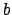to be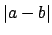. Assume for the rest of this section that we only consider valuations that satisfy the triangle inequality.

Lemma 16.1.2   A field with the topology induced by a valuation is a , i.e., the operations sum, product, and reciprocal are continuous.

Proof. For example (product) the triangle inequality implies that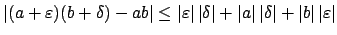is small when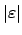and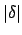are small (for fixed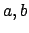).Lemma 16.1.3   Suppose two valuationsandon the same fieldinduce the same topology. Then for any sequence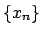inwe have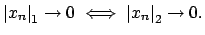Proof. It suffices to prove that if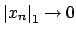then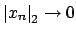, since the proof of the other implication is the same. Let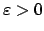. The topologies induced by the two absolute values are the same, so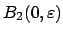can be covered by open balls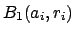. One of these open balls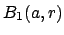contains 0. There is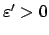such that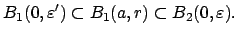Since, there exists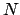such that for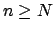we have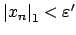. For such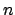, we have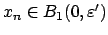, so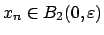, so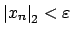. Thus.Proposition 16.1.4   If two valuationsandon the same field induce the same topology, then they are equivalent in the sense that there is a positive real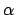such that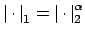.

Proof. If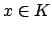and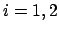, then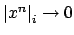if and only if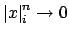, which is the case if and only if. Thus Lemma 16.1.3 implies that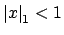if and only if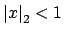. On taking reciprocals we see that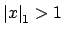if and only if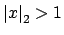, so finally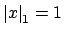if and only if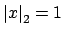.

Let now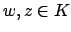be nonzero elements with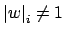and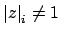. On applying the foregoing to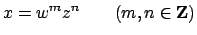we see that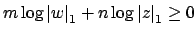if and only if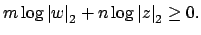Dividing through by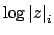, and rearranging, we see that for every rational number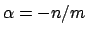,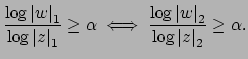Thus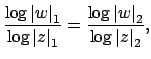so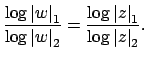Since this equality does not depend on the choice of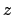, we see that there is a constant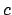(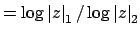) such that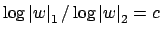for all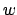. Thus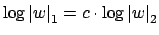, so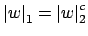, which implies thatis equivalent to.William Stein 2004-05-06